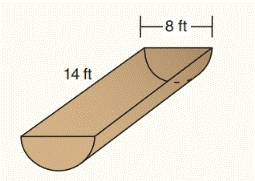Chapter 9.CR, Problem 14CR### Elementary Geometry for College St...

6th Edition
Daniel C. Alexander + 1 other
ISBN: 9781285195698

#### Solutions

Chapter
Section### Elementary Geometry for College St...

6th Edition
Daniel C. Alexander + 1 other
ISBN: 9781285195698
Textbook Problem
5 views

# a) For the through in the shape of half-cylinder, find the volume of water it will hold (Use π ≈ 3.14 and disregard the thickness)b) If the trough is to be painted inside and out, find the number of square feet to be painted (Use π ≈   3.14 . )To determine

(a)

To find:

The volume of water it will hold.

Explanation

Formula used:

Volume of half-cylinder:

V=12πr2h

Given:

For the through in the shape of half-cylinder as shown the below figure,

Use π = 3.14

Calculation:

Let V=12πr2h(1)

The diameter of half cylinder is,

d=8

To determine

(b)

To find:

The number of square feet to be painted.

### Still sussing out bartleby?

Check out a sample textbook solution.

See a sample solution

#### The Solution to Your Study Problems

Bartleby provides explanations to thousands of textbook problems written by our experts, many with advanced degrees!

Get Started

#### (a) 53=125,solog= (b) log525=2,so=

Precalculus: Mathematics for Calculus (Standalone Book)

#### g(x)=2(x1)5/2

Applied Calculus for the Managerial, Life, and Social Sciences: A Brief Approach

#### Evaluate the expression sin Exercises 116. 33

Finite Mathematics and Applied Calculus (MindTap Course List)

#### Evaluate the integral. 13. x29x3dx

Single Variable Calculus: Early Transcendentals

#### Solve: x(x+5)=1.

College Algebra (MindTap Course List)